# Functions, Relations and Trigonometry | The Ultimate NESA Maths Reference Sheet Guide

Make the most of NESA Maths Reference Sheet and test your maths skills with these sample questions and explanations for functions, relations and trigonometry!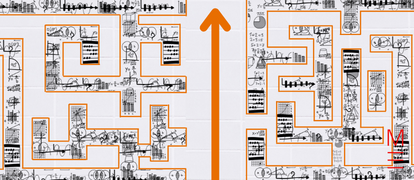The NESA Maths Reference Sheet is a great resource… if you know how to use it! Navigate functions (including trigonometric ones!) and relations with our Ultimate NESA Maths Reference Sheet Guide.

While memorisation has its place in learning, Matrix recommends that students learn to derive their responses and learn how to apply these formulae correctly. As a bonus, we’ve included a nifty HSC Maths Cheatsheet for you to download and print out!A free pocket-sized Maths handbook, so you're prepared.

All the key Maths formulas you need to revise, in one foldable cheatsheet.

Click on the following formulas to see what they mean and apply them to a practice question!

 Functions $$x=\frac{-b± \sqrt{b^2-4ac}}{2a}$$ $$\text{For} \ ax^3 + bx^2 +cx + d = 0 ;$$ Sum of roots: $$α+β+γ = \text{ } – \frac{b}{a}$$ Sum in Pairs:  $$αβ+αγ+βγ = \frac{c}{a}$$ Product of roots: $$αβγ = -\frac{d}{a}$$

## Functions

 Use Formula Variables Solve quadratic equations $$x=\frac{-b± \sqrt{b^2-4ac}}{2a}$$ a, b and c are the coefficients of a quadratic equation in the form:$$ax^2+bx+c=0$$

Example 12:

Consider the following equation: $$-2y^2+3y+1=0$$

Find all the solutions of $$y$$.

Solution 12:

 Even though the above equation uses $$y$$ as a variable instead of $$x$$, the quadratic formula works the same way. \begin{align*} y &= \frac{-b± \sqrt{b^2-4ac}}{2a} \\ & \text{where a, b and c are the coefficients of a quadratic equation:} -2y^2+3y+1=0 \\ \end{align*} Hence, substitute $$a=-2$$, $$b=3$$ and $$c=1$$ into $$y=\frac{-b± \sqrt{b^2-4ac}}{2a}$$: \begin{align*} y &= \frac{-3± \sqrt{3^2-4 \times (-2) \times 1}}{2 \times (-2)} \\ &= \frac{-3± \sqrt{9+8}}{-4} \\ &= \frac{-3± \sqrt{17}}{-4} \\ &= \frac{3± \sqrt{17}}{4} \\ ∴ y &= \frac{3+ \sqrt{17}}{4} \text{  or  } y = \frac{3- \sqrt{17}}{4}\\ \end{align*}

 Use Formula Variables Cubic roots \begin{align*} \text{For} \ ax^3 + bx^2 +cx + d &= 0: \\ α+β+γ &= – \frac{b}{a}\\ αβ+αγ+βγ &= \frac{c}{a}\\ \text{and } αβγ &= -\frac{d}{a}\\ \end{align*} α, β and γ are roots of a cubic equation in the form: $$ax^3 + bx^2 +cx + d = 0$$

Example 13:

$$x=1$$ and $$x=3$$ are two solutions to the monic equation: $$ax^3 + bx^2 +cx + d = 0$$

Given that the product of the roots is -6, find the coefficients $$a,b,c$$ of this cubic polynomial.

Solution 13:

 The question states that the equation is monic. This means that, a=1. \begin{align*} \text{Let } α, β, γ \text{ be roots of the given cubic equation} \\ \\ αβγ &= -\frac{d}{a} \\ &= -d \text{  (since a=1)} \\ &= -6 \text{  (product of the roots is -6)}\\ ⇒ d &=6\\ \\ α+β+γ &= – \frac{b}{a}\\ ⇒ \text{Equation 1: } α+β+γ &= -b \text{  (since a=1)} \\ \\ αβ+αγ+βγ &= \frac{c}{a}\\ ⇒ \text{Equation 2: } αβ+αγ+βγ &= c \text{  (since a=1)} \end{align*} It’s given that $$x=1$$ and $$x=3$$ are solutions to the cubic equation, $$x=1$$ and $$x=3$$ are two roots of the equation. Hence, substitute $$α=1$$ and $$β=3$$ into $$αβγ = -6$$: \begin{align*} (1)(3)γ &= -6 \\ ∴ γ &= -2 \\ \end{align*} To find the coefficients b and c (we’ve already found $$a=1$$ and $$d=6$$): Substitute $$α=1$$, $$β=3$$ and $$γ=-2$$ into equation 1: $$α+β+γ = -b$$ and equation 2: $$αβ+αγ+βγ = c$$: \begin{align*} \text{Equation 1: } 1+3+-2 &= -b  \\ ∴b &= -2  \\ \\ \text{Equation 2: } (1)(3)+(1)(-2)+(3)(2) &= c \\ 3+(-2)+6 &= c \\ ∴ c &= 7 \\ \end{align*} Therefore, the coefficients of the equation $$ax^3 + bx^2 +cx + d = 0$$ are $$a=1$$, $$b=-2$$,$$c=7$$ and $$d=6$$.

### Relations

 Use Formula Explanation Cartesian equation of a circle $$(x-h)^2+(y-k)^2=r^2$$ Cartesian equation of a circle centred at (h, k) with radius = r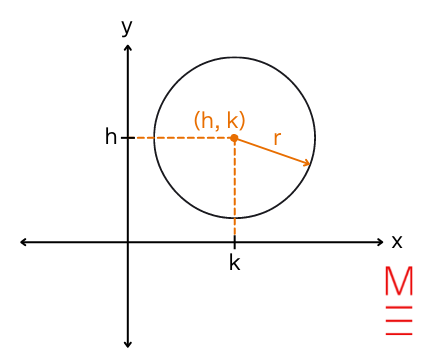Example 14:

The centre of a circle lies at the intersection of two lines: $$y = x$$ and $$y = 2x – 4$$

Given that the area of this circle is $$16π$$ units2, find the Cartesian equation of this circle.

Solution 14:

 To find the centre of the circle, first we simultaneously solve the equations $$y = x$$ and $$y = 2x – 4$$ to find their point of intersection.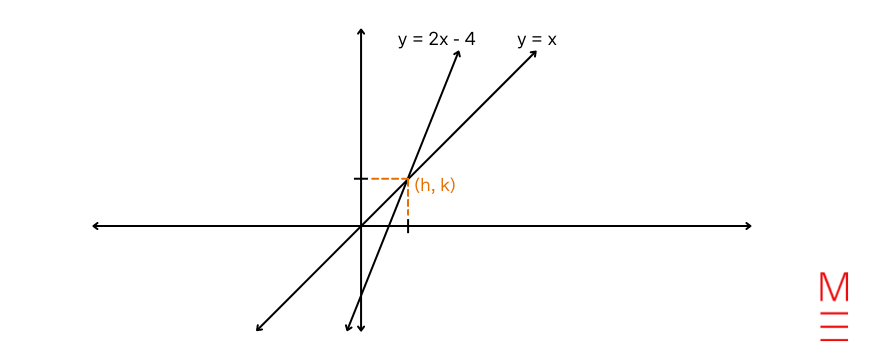\begin{align*} \text{Substitute } y &= x \text{ into } y = 2x – 4 : \\ x &= 2x-4 \\ ∴ x &= 4 \\ \\ \text{Substitute } x &= 4 \text{ into } y = 2x – 4 : \\ y &= 2(4) – 4 \\ ∴ y &= 4 \\ \\ ⇒ \text{Centre of circle (h, k) } & = (4, 4) \\ \end{align*} To find the radius r of the circle, we can use the information that the area of this circle is $$16π$$ units2. The formula for the area of a circle is: $$A = \pi r^2$$ \begin{align*} \text{Substitute } A & = 16 \pi \text{ into } A = \pi r^2 : \\ 16 \pi &= \pi r^2 \\ 16 &= r^2 \\ ∴r &= 4 \ \ (r > 0)\\ \end{align*} The equation of the circle is: $$(x-h)^2+(y-k)^2=r^2$$ where $$(h, k)$$ = $$(4, 4)$$ and $$r = 4$$ \begin{align*} (x-4)^2+(y-4)^2 & =4^2 \\ ⇒ \text{Cartesian equation of the circle: } (x-4)^2+(y-4)^2 & =16 \\ \end{align*}

## Logarithmic and Exponential Functions

 Formula Application $$\text{log}_a a^x = x = a^{\text{log}_a x}$$ Simplifying and manipulating expressions. $$\text{log}_a x = \frac{\text{log}_b x}{\text{log}_b a}$$ Most calculators can calculate $$\text{log}_{10}$$ and $$\text{ln}$$. To input logarithms without base 10 or e, you can use this formula convert your logarithm into an expression involving logarithms with only base 10 or e. e.g. $$\text{log}_8 9 = \frac{\text{log}_{10} 9}{\text{log}_{10} 8} = \frac{ \text{ln}9}{\text{ln}8}$$ $$\text{log}_2 5 = \frac{\text{log}_{10} 5}{\text{log}_{10} 2}= \frac{ \text{ln}5}{\text{ln}2}$$ $$a^x = e^{x\text{ln}a}$$ To integrate expressions in the form $$a^x$$: \begin{align*} & \int a^x \ dx \\ &= \int e^{x\text{ln}a} \ dx \\ &= \frac{1}{\text{ln}a} \ e^{x\text{ln}a} + c \\ &= \frac{1}{\text{ln}a} \ a^x + c\\ \end{align*} Review your knowledge of integration by substitution here.

## Trigonometric Functions

 Use Formula Explanation Trigonometric functions \begin{align*} \text{sin}A = \frac{\text{opp}}{\text{hyp}} \\ \text{cos}A = \frac{\text{adj}}{\text{hyp}} \\ \text{tan}A = \frac{\text{opp}}{\text{adj}} \\ \end{align*}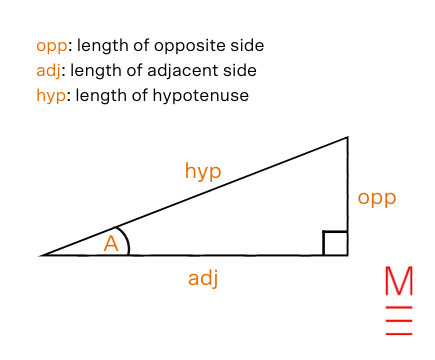Example 15:

Given the below right-angle triangle, find the value of the following trigonometric expressions:

a) sinA

b) cos(90°-A)

c) tan90°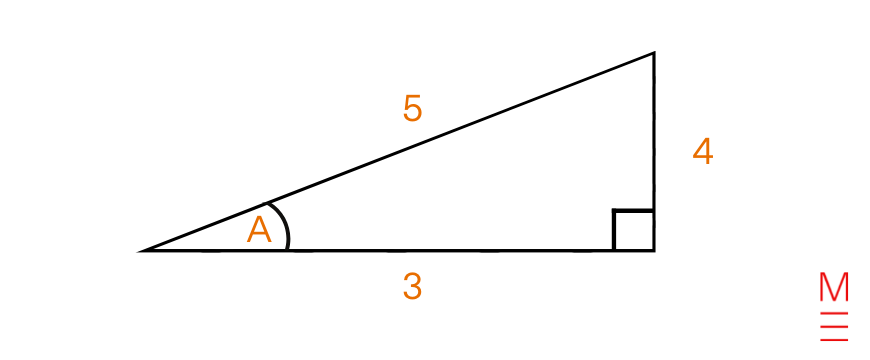Solution 15:

 a) sinA = $$\frac{4}{5}$$ b) cos(90°-A) = sinA = $$\frac{4}{5}$$ Alternatively, recognise that (90°-A) is an angle in the triangle.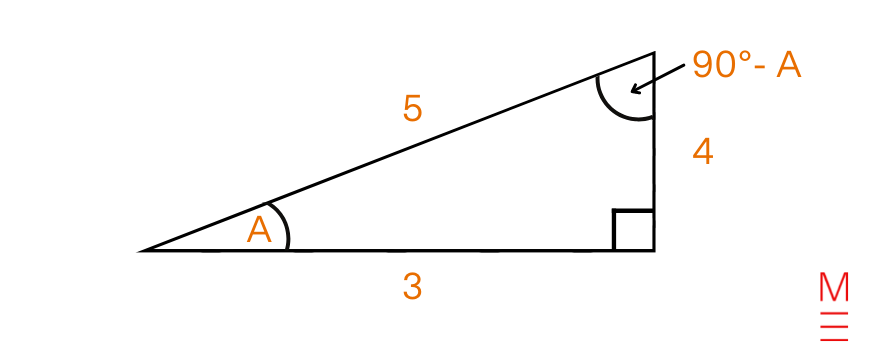Hence, cos(90°-A) = $$\frac{\text{adj}}{\text{hyp}}$$ = $$\frac{4}{5}$$ c) tan90° = undefined As we can see in this right angle triangle, the length opposite 90° is the hypotenuse. Hence, the expression $$\text{tan}90° = \frac{\text{opp}}{\text{adj}}$$ is undefined.

 Use Formula Explanation Area of a triangle $$A= \frac{1}{2}ab\text{sin}C$$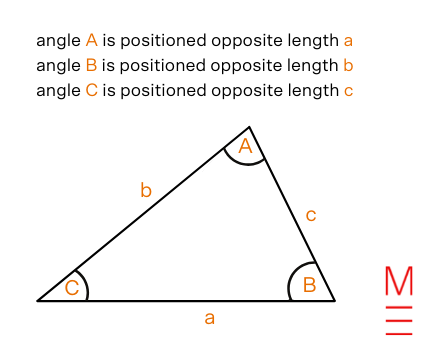Example 16:

Given that the following triangle has an area of $$4 \sqrt{3}$$ cm2, find the length x.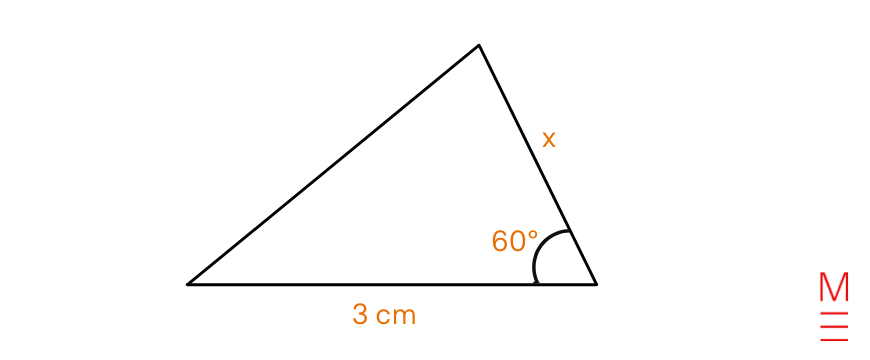Solution 16:

 Substitute $$A= 4 \sqrt{3}$$, $$a=3$$, $$b=x$$ and $$C=60°$$ into $$A= \frac{1}{2}ab\text{sin}C$$: \begin{align*} 4 \sqrt{3} &= \frac{1}{2} (3)(x)\text{sin}60° \\ 8 \sqrt{3} &= (3)(x)\text{sin}60° \\ 8 \sqrt{3} &= (3)(x)\frac{\sqrt{3}}{2} \\ x &= \frac{ 8 \sqrt{3} \times 2}{3\sqrt{3}}\\ ∴x &= \frac{16}{3} \text{ cm}\\ \end{align*}

 Use Formula Explanation Sine rule $$\frac{a}{\text{sin}A} = \frac{b}{\text{sin}B} = \frac{c}{\text{sin}C}$$Cosine rule $$c^2=a^2+b^2-2ab\text{cos}C$$ This equation can also be rearranged as: $$\text{cos}C = \frac{a^2+b^2-c^2}{2ab}$$

Example 17:

Find length x. Giver your answer to the nearest 3 decimal places.

The diagram is not to-scale.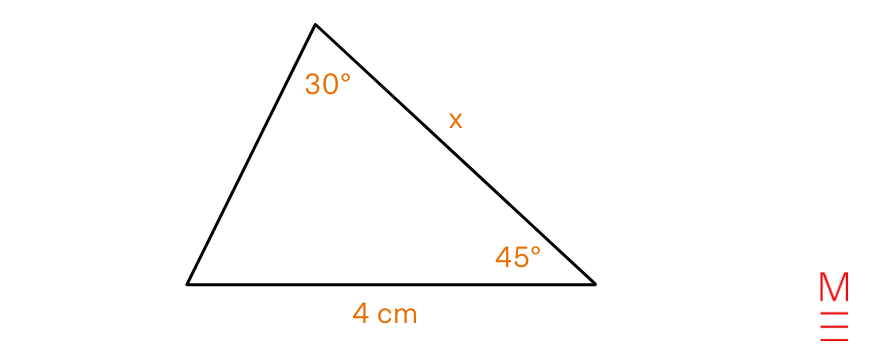Solution 17:

 In order to use the sine rule to find x, we need to know the angle θ that is opposite length x.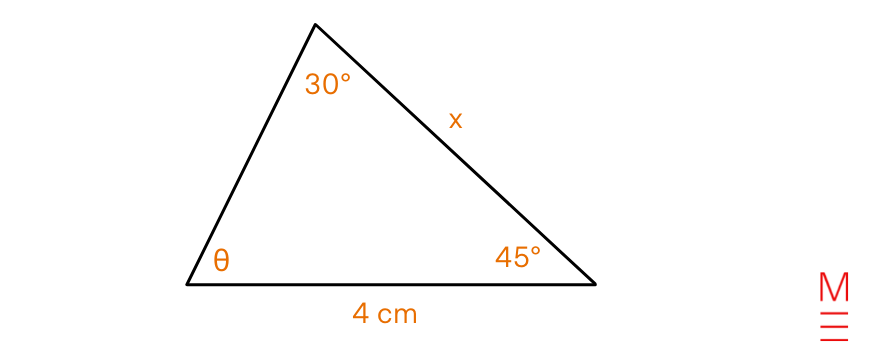\begin{align*} θ + 45° + 30° &= 180°  \text{  (angle sum of a triangle)}\\ ∴θ &= 105° \\ \end{align*} Thus, we can use the sine rule: Substitute $$a=4$$, $$A=30°$$, $$b=x$$, $$B=θ=105°$$ into $$\frac{a}{\text{sin}A} = \frac{b}{\text{sin}B}$$: \begin{align*} \frac{4}{\text{sin}30°} &= \frac{x}{\text{sin}105°} \ \text{ (sine rule)}\\ x &= \frac{4 \times \text{sin}105°}{\text{sin}30°} \\ ∴ x &= 7.727 \text{ cm     (to the nearest 3 decimal places)}  \\ \end{align*}

 Use Formula Explanation Arc length of a sector (using θ in radians: $$\pi ^c = 180°$$) $$l=r \theta$$ \begin{align*} l&= \text{arc length}\\ \theta &= \text{angle of sector in radians}\\ r &= \text{radius}\\ \end{align*}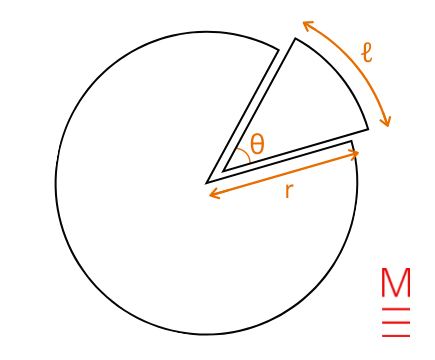Area of a sector (using θ in radians: $$\pi ^c = 180°$$) $$A= \frac{1}{2} r^2 \theta$$

Example 18:

a) Sal is fencing a paddock for his sheep. He wants to make the paddock the shape of a sector with a radius of 6 metres. If he has $$12 + 2π$$ metres of fencing, what is the angle of the largest sector he can fully enclose? Give your answer as an exact value in radians.

b) Hence, find the maximum area of Sal’s paddock.

Solution 18 a):

 The following diagram illustrates the paddock.The perimeter of the sector: $$P = r+r+l.\text{ }$$Since Sal is limited to $$(12 + 2π)$$ metres of fencing, substitute $$P =12 + 2π$$ and $$r=6$$ (given).\begin{align*} 12 + 2π &= 6 + 6 + l \\ l &= 2π \\ \end{align*}Hence, the arc length of the largest sector Sal can enclose is $$2π$$. However, this is not our final answer as we are required to find the angle of the largest sector. Substitute $$l = 2π$$ and $$r=6$$ into $$l=r \theta$$:\begin{align*} 2π &= 6 \times \theta \\ \theta &= \frac{π}{3} \\ ∴ \text{Angle of the largest sector} &= \frac{π}{3}^c \\ \end{align*}

Solution 18 b):

 We are given that the radius of the sector is 6 metres and in part a), we found that the largest possible angle of the sector is $$frac{π}{3}^c$$. So, to find the largest area of the sector, substitute $$r=6$$ and $$\theta=frac{π}{3}^c$$ into $$A= \frac{1}{2} r^2 \theta$$: \begin{align*} A &= \frac{1}{2} \times 6^2 \times \frac{π}{3} \\ A &= 6 \pi \\ ∴ \text{Maximum area of the sector} &= 6 \pi \text{ m}^2\\ \end{align*}

### Trigonometric identities

 Identity Variables $$\text{sec}A = \frac{1}{\text{cos}A}, \ \text{cos}A ≠0$$ Angle $$A$$ can take any variable (except for where exceptions are specifically noted) and the identity will hold true. $$\text{cosec}A = \frac{1}{\text{sin}A}, \ \text{sin}A ≠0$$ $$\text{cot}A = \frac{\text{cos}A}{\text{sin}A}, \ \text{sin}A ≠0$$ $$\text{cos}^2x+\text{sin}^2x=1$$

Example 19:

Prove that: $$\text{tan}θ\text{sin}θ + \text{cos}θ = \text{sec}θ$$

Solution 19:

 \begin{align*} LHS &= \text{tan} \theta \text{sin} \theta + \text{cos} \theta \\ \text{Since } \text{tan} \theta = \frac{ \text{sin} \theta }{ \text{cos} \theta }, LHS &= \frac{ \text{sin} \theta }{ \text{cos} \theta } \times \text{sin} \theta + \text{cos} \theta \\ &= \frac{ \text{sin}^2 \theta }{ \text{cos} \theta } + \frac{ \text{cos}^2 \theta }{ \text{cos} \theta } \\ &= \frac{ \text{sin}^2 \theta + \text{cos}^2 \theta }{ \text{cos} \theta } \\ \text{Since }  \text{sin}^2 \theta + \text{cos}^2 \theta=1, &= \frac{1}{ \text{cos} \theta } \\ &=  \text{sec} \theta \\ &= RHS \\ ∴ \text{tan} \theta \text{sin} \theta + \text{cos} \theta = \text{sec} \theta \\ \end{align*}

### Compound angles

Expanding a sum of angles in a trigonometry function

 Identity Variables $$\text{sin}(A+B)=\text{sin}A\text{cos}B+\text{cos}A\text{sin}B$$ $$A, \ B ∈ R$$ Angle $$A$$ and $$B$$ can take any value (they can be equal or unequal) and the identity will hold true. $$\text{cos}(A+B)=\text{cos}A\text{cos}B-\text{sin}A\text{sin}B$$ $$\text{tan}(A+B)=\frac{\text{tan}A+\text{tan}B}{1-\text{tan}A\text{tan}B}$$

Example 20:

Find the exact value of $$cos75º$$.

Solution 20:

 \begin{align*} \text{cos}75º &= \text{cos}(45+30)º \\ \text{Since } \text{cos}(A+B)=\text{cos}A\text{cos}B-\text{sin}A\text{sin}B \\ \text{cos}(45+30)º &= \text{cos}45\text{cos}30-\text{sin}45\text{sin}30 \\ &= \frac{ \sqrt{2}}{2} \times \frac{ \sqrt{3}}{2} – \frac{ \sqrt{2}}{2} \times \frac{1}{2} \\ &= \frac{ \sqrt{6}}{4} – \frac{ \sqrt{2}}{4} \\ ∴ \text{cos}75º &= \frac{ \sqrt{6} – \sqrt{2}}{2} \\ \end{align*}

T-formula

 Formula Explanation \begin{align*} \text{If } t=\text{tan} \frac{A}{2} \text{ then } \text{sin}A &= \frac{2t}{1+t^2}\\ \text{cos}A &= \frac{1-t^2}{1+t^2}\\ \text{tan}A &= \frac{2t}{1-t^2}\\ \end{align*} $$A ∈ R$$ Substitute sinA, cosA or tanA with these ‘t-values’. That way you won’t have to deal with trigonometric functions, only algebraic expressions involving t. Once you have simplified your expression involving t, often you will need to substitute $$t=\text{tan} \frac{A}{2}$$ to find the values of $$A$$.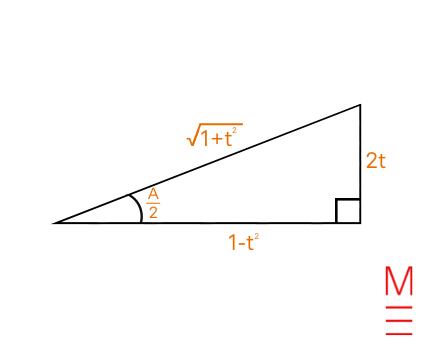Note: When A = 180°, $$t=\text{tan} \frac{A}{2} = \text{tan}90$$ is undefined. That means that you will need to separately check whether A = 180° it is a solution when you use the t-formula. See Example 21 below.

Example 21:

Given $$2\text{cos}2B – \text{sin}2B = 1$$, solve for $$B$$, $$0 \le B \le 180°$$:

If necessary, give your answers to the nearest 2 decimal places.

Solution 21:

 \begin{align*} \text{Let } t &= \text{tan}B \\ ⇒ \text{sin}2B &= \frac{2t}{1+t^2}\\ \text{cos}2B &= \frac{1-t^2}{1+t^2}\\ \text{tan}2B &= \frac{2t}{1-t^2}\\ \\ \text{Given that }2\text{cos}2B – \text{sinB}2B  &= 1 \\ ⇒ 2 \times \frac{1-t^2}{1+t^2} – \frac{2t}{1+t^2}  &= 1 \\ (1+t^2) \times (2 \times \frac{1-t^2}{1+t^2} – \frac{2t}{1+t^2}) &= (1+t^2) \times 1 \\ 2 (1-t^2) – 2t &= 1+t^2 \\ 2 – 2t^2 – 2t &= 1+t^2 \\ -3t^2 – 2t + 1 &= 0 \\ 3t^2 + 2t – 1 &= 0 \\ (3t-1)(t+1) &= 0 \\ 3t-1 &= 0 \text{   or   } t+1=0 \\ t &= \frac{1}{3} \text{   or   } t=-1 \\ ⇒ \text{tan}B &= \frac{1}{3} \text{   or   } \text{tan}B=-1 \\ ∴ B &= \text{tan}^{-1} \frac{1}{3} \text{   or   } B = 180 + {tan}^{-1}(-1)   \text{   (since 0

Manipulating a product of trigonometric functions into a sum of trigonometric functions

 Identity Variables $$\text{cos}A\text{cos}B=\frac{1}{2}[\text{cos}(A-B)+\text{cos}(A+B)]$$ Angle $$A$$ and $$B$$ can take any variable (they can be equal or unequal) and the identity will hold true. $$\text{sin}A\text{sin}B=\frac{1}{2} [\text{cos}(A-B) -\text{cos}(A+B)]$$ $$\text{sin}A\text{cos}B = \frac{1}{2} [\text{sin}(A+B)+\text{sin}(A-B)]$$ $$\text{cos}A\text{sin}B=\frac{1}{2}[\text{sin}(A+B)-\text{sin}(A-B)]$$

Example 22:

Find the exact value of the product of $$\text{cos}15º$$ and $$\text{sin}45º$$.

Solution 22:

 \begin{align*} \text{Since } \text{cos}A\text{sin}B & =\frac{1}{2}[\text{sin}(A+B)-\text{sin}(A-B)] \\ ⇒ \text{cos}15º\text{sin}45º &= \frac{1}{2}[\text{sin}(15+45)-\text{sin}(15-45)] \\ &= \frac{1}{2}[\text{sin}(60)-\text{sin}(-30)] \\ &= \frac{1}{2}[\frac{ \sqrt{3}}{2}+ \frac{1}{2} ] \\ ∴\text{cos}15º\text{sin}45º &= \frac{\sqrt{3}+1}{4} \end{align*}

Double angle identities

 Identity Variables $$\text{sin}^2nx=\frac{1}{2}(1-\text{cos}2nx)$$ $$x, \ n ∈ R$$ $$\text{cos}^2nx=\frac{1}{2}(1+\text{cos}2nx)$$

Example 23:

Evaluate $$2(\text{sin}22.5º)^2$$. Give your answer in exact form.

Solution 23:

 \begin{align*} \text{Since } \text{sin}^2nx &=\frac{1}{2}(1-\text{cos}2nx) \\ ⇒ 2(\text{sin}22.5º)^2 &= 2 \times \frac{1}{2}(1-\text{cos}45º) \\ &= 1- \frac{ \sqrt{2}}{2} \\ &=  \frac{ 2- \sqrt{2}}{2}\\ ∴2(\text{sin}22.5º)^2 &= \frac{ 2- \sqrt{2}}{2} \\ \end{align*}